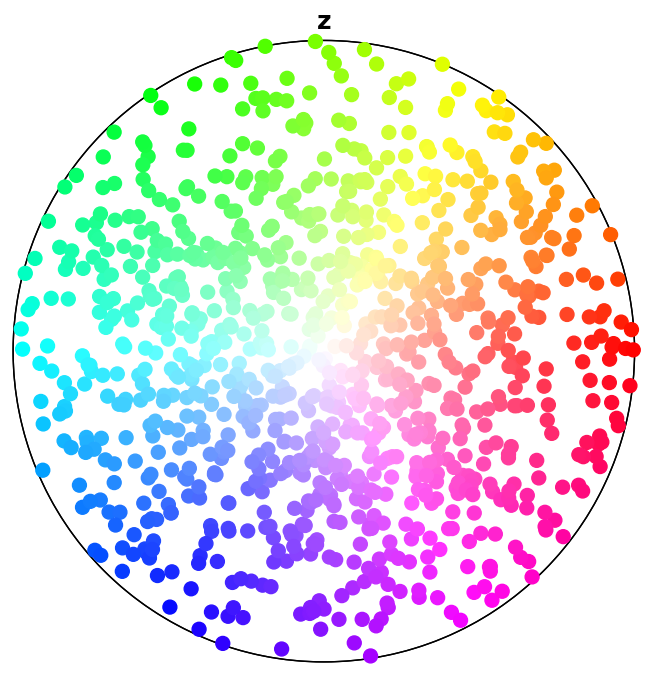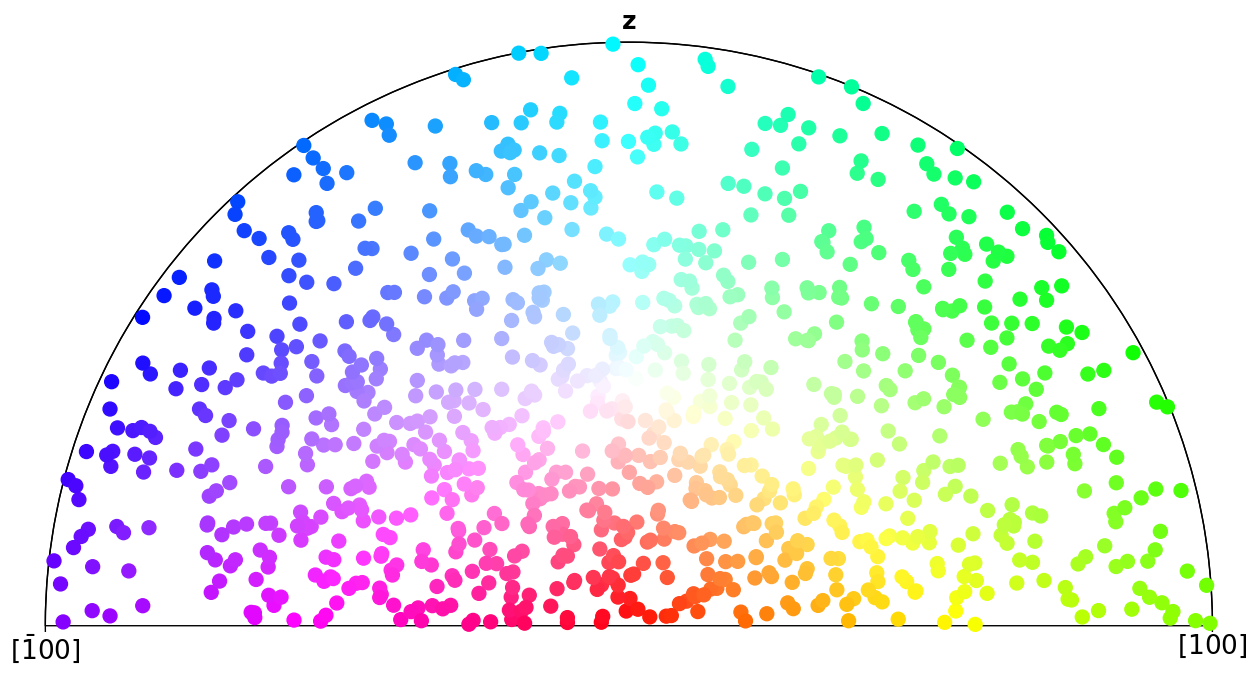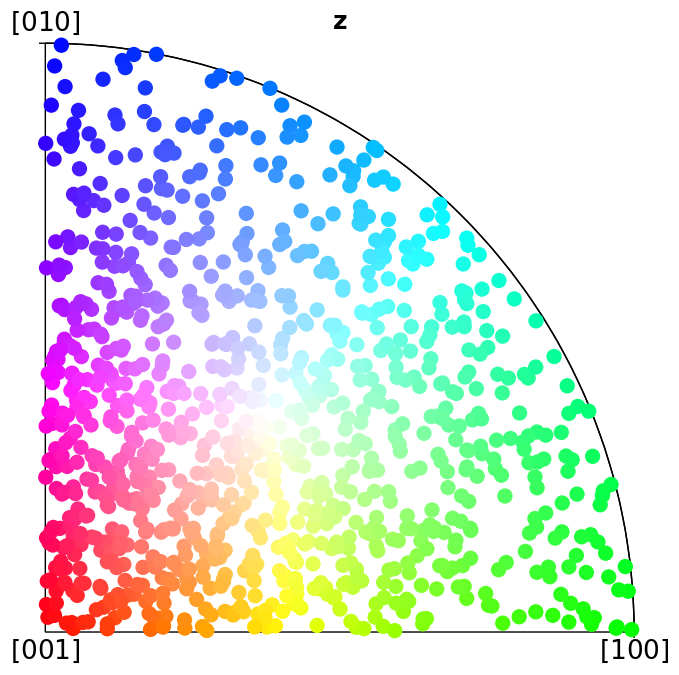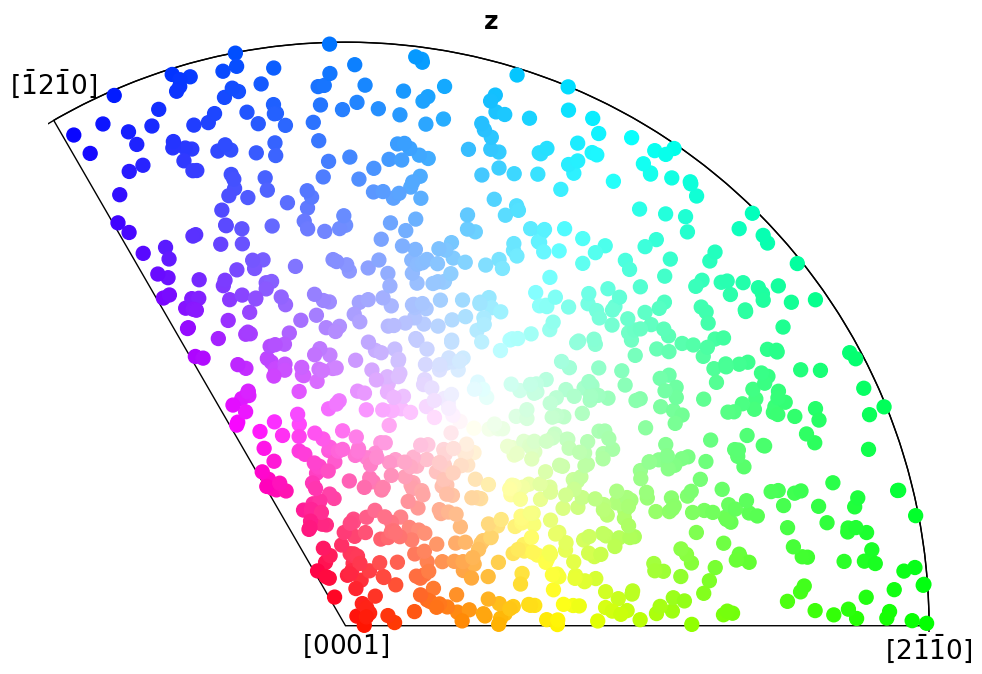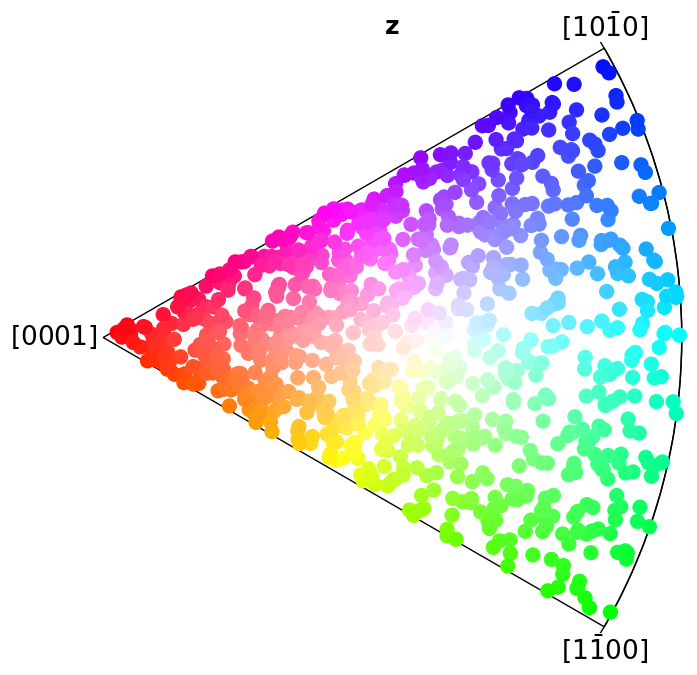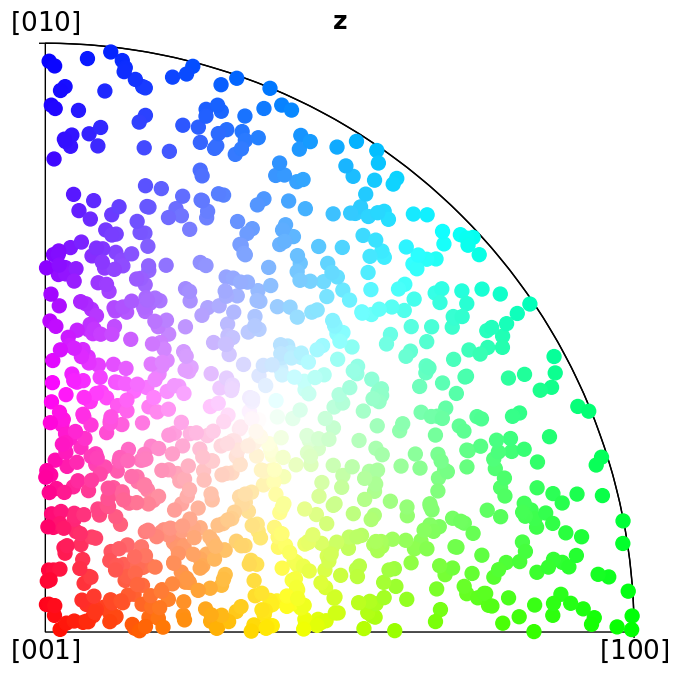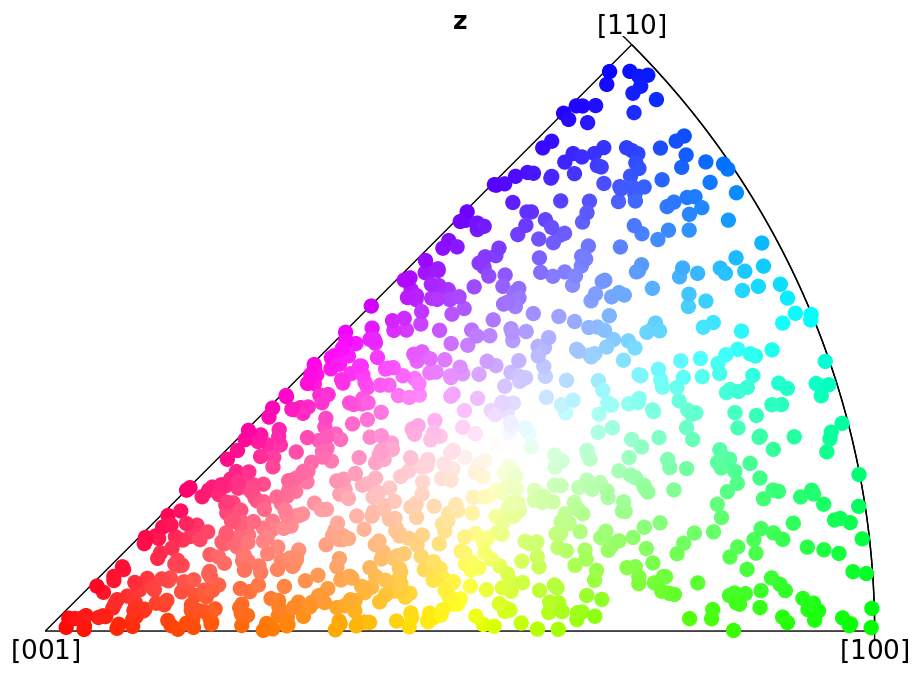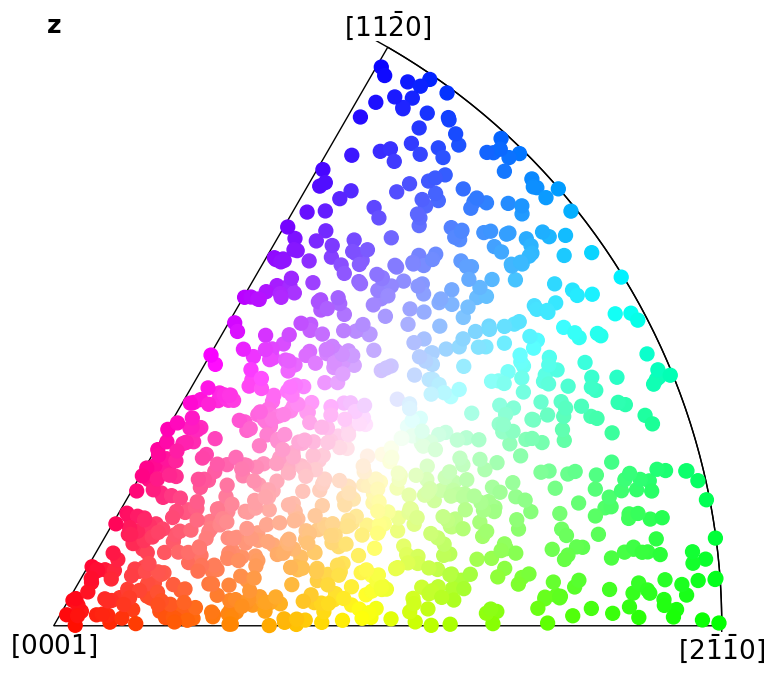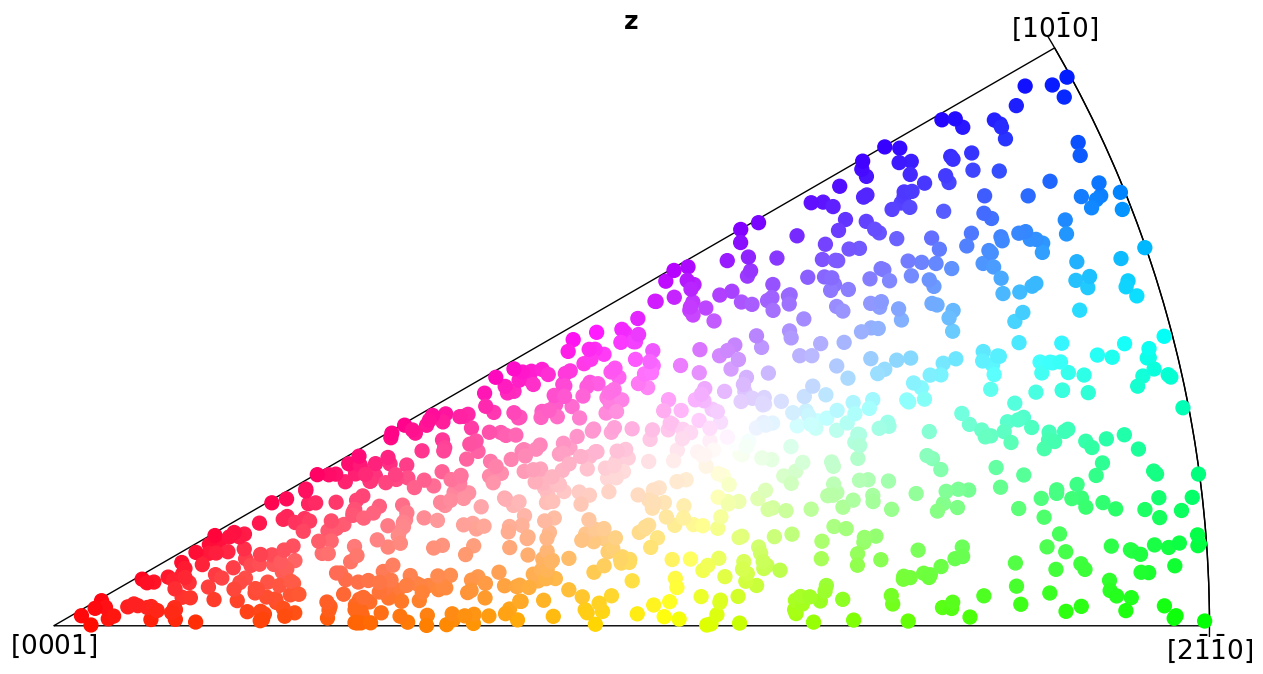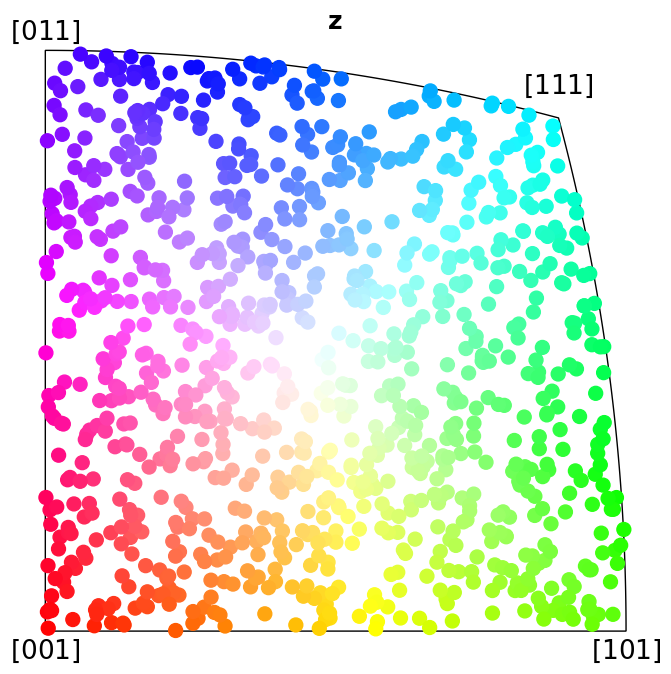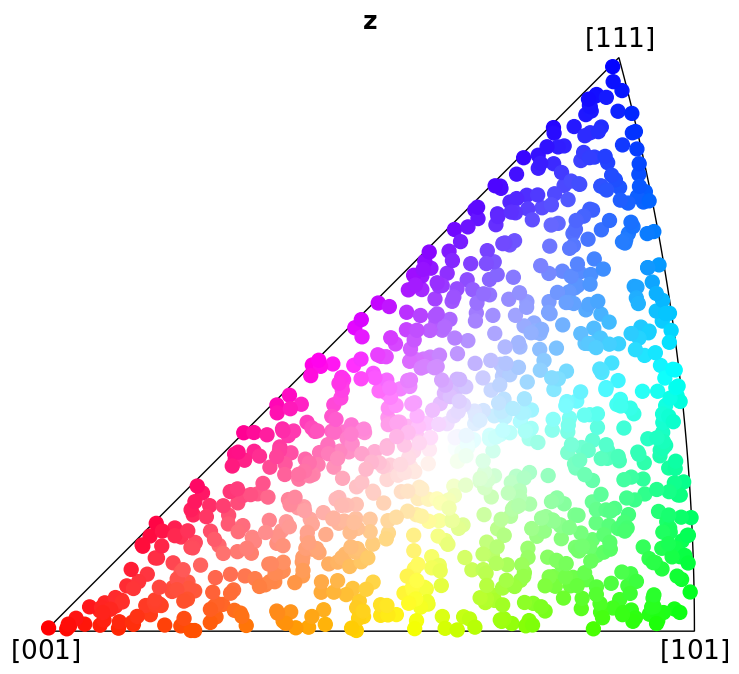# Inverse pole figures#

In this tutorial we will express in which crystal direction $$\left<uvw\right>$$ any sample direction X, Y, Z, or any Vector3d, is parallel to. Formally, this so-called inverse pole figure (IPF) shows the directional distribution of a set of reference vectors in terms of a fixed crystallographic frame . By assigning a unique colour to each crystal direction in a symmetry’s fundamental sector (the IPF), we can colour orientations .

While orix has descriptions for all 32 crystallographic point groups, so far only the fundamental sectors of the eleven Laue group symmetries have been defined. Thus, the Laue class (in the left and center column in the table below) of the point groups (center and right column) will be used when plotting the IPF and colouring orientations.

Schoenflies

Laue

Non-centrosymmetric

Ci

$$\bar{1}$$

1

C2h

2/m

2, m

D2h

mmm

222, 2mm

S6

$$\bar{3}$$

3

D3d

$$\bar{3}$$m

32, 3m

C4h

4/$$m$$

4, $$\bar{4}$$

D4h

4/$$mmm$$

422, $$\bar{4}2m$$, 4$$mm$$

C6h

6/$$m$$

6, $$\bar{6}$$

D6h

6/mmm

622, $$\bar{6}2m$$, 6mmm

Th

$$m\bar{3}$$

23

Oh

$$m\bar{3}m$$

432, $$\bar{4}3m$$

Only the colour keys used in EDAX TSL OIM Analysis are supported, via the IPFColorKeyTSL.

The IPF functionality in the excellent software MTEX and the two above referenced works have been the basis for the IPF functionality in orix.

:

%matplotlib inline

import matplotlib.pyplot as plt
import numpy as np

from orix import plot, sampling
from orix.crystal_map import Phase
from orix.quaternion import Orientation, symmetry
from orix.vector import Vector3d

# We'll want our plots to look a bit larger than the default size
new_params = {
"figure.facecolor": "w",
"figure.figsize": (20, 7),
"lines.markersize": 10,
"font.size": 15,
"axes.grid": True,
}
plt.rcParams.update(new_params)


## Minimal example of plotting the IPF colour key#

:

plot.IPFColorKeyTSL(symmetry.D3d).plot()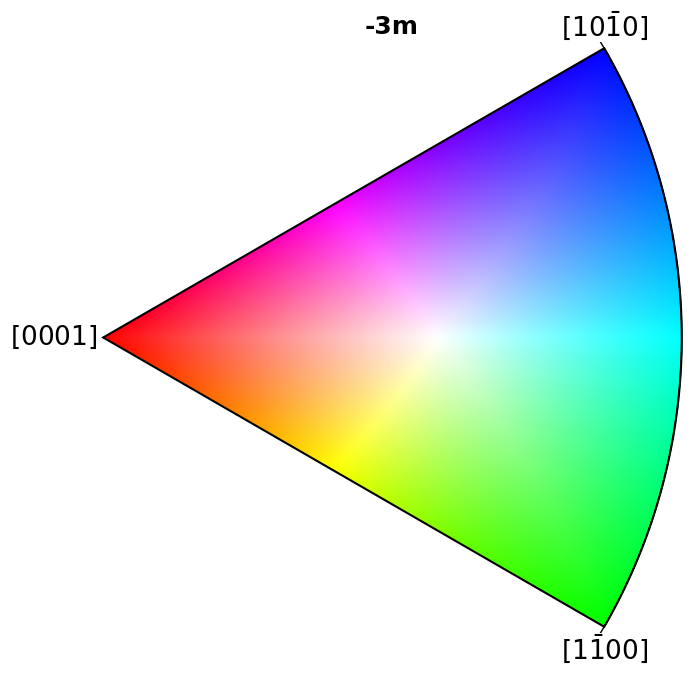## Plot colour keys#

Here we plot the colour keys for the eleven Laue groups. An example demonstrating how an IPF color key can be added to a custom plot is shown in the Crystal map tutorial.

:

pg_laue = [
symmetry.Ci,
symmetry.C2h,
symmetry.D2h,
symmetry.S6,
symmetry.D3d,
symmetry.C4h,
symmetry.D4h,
symmetry.C6h,
symmetry.D6h,
symmetry.Th,
symmetry.Oh,
]

:

for pg in pg_laue:
ipfkey = plot.IPFColorKeyTSL(pg)
ipfkey.plot()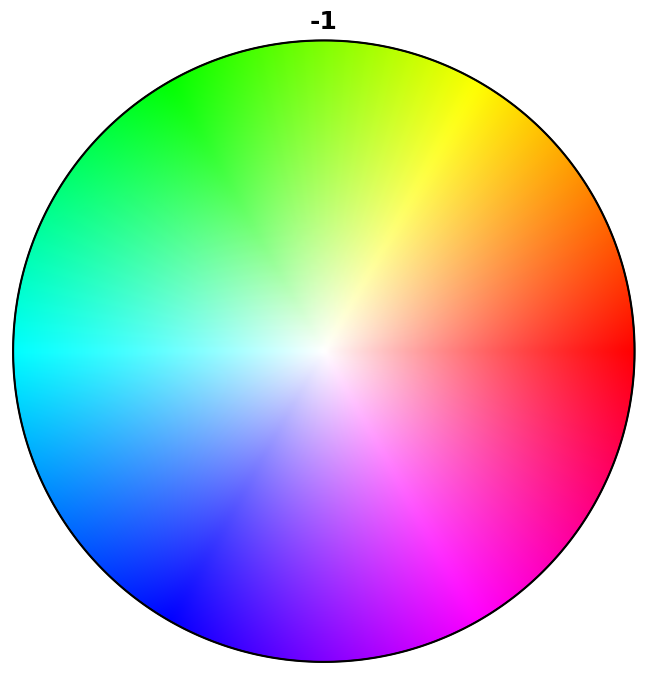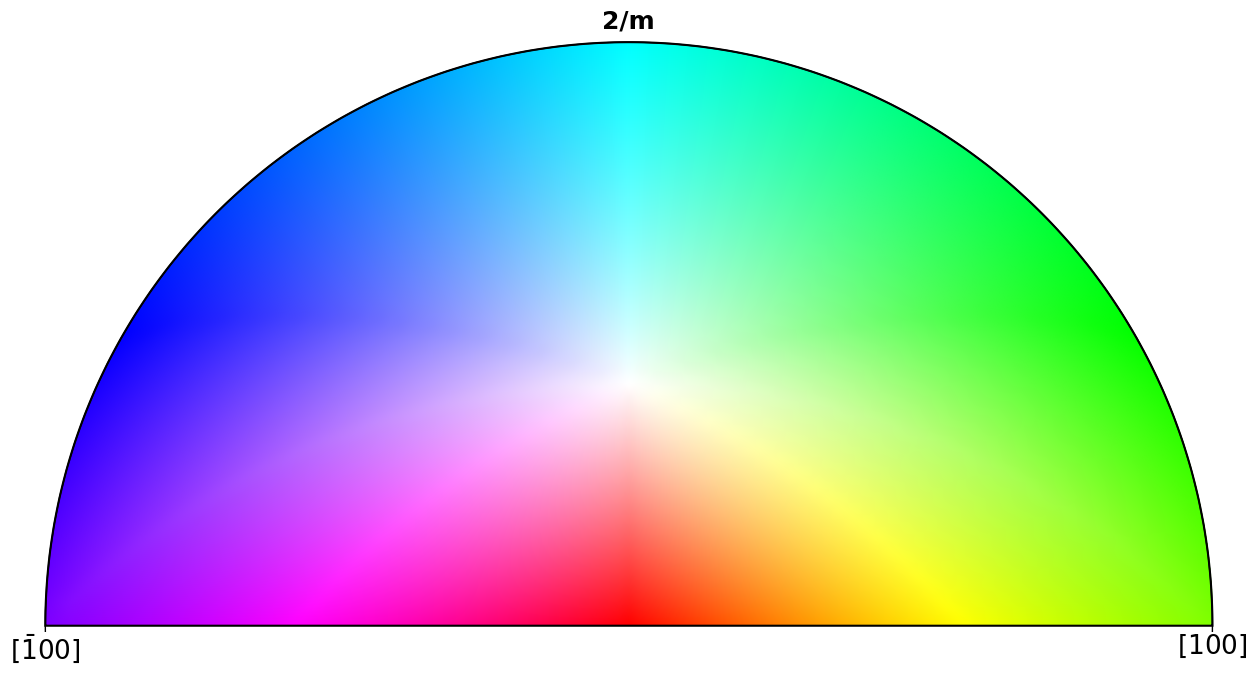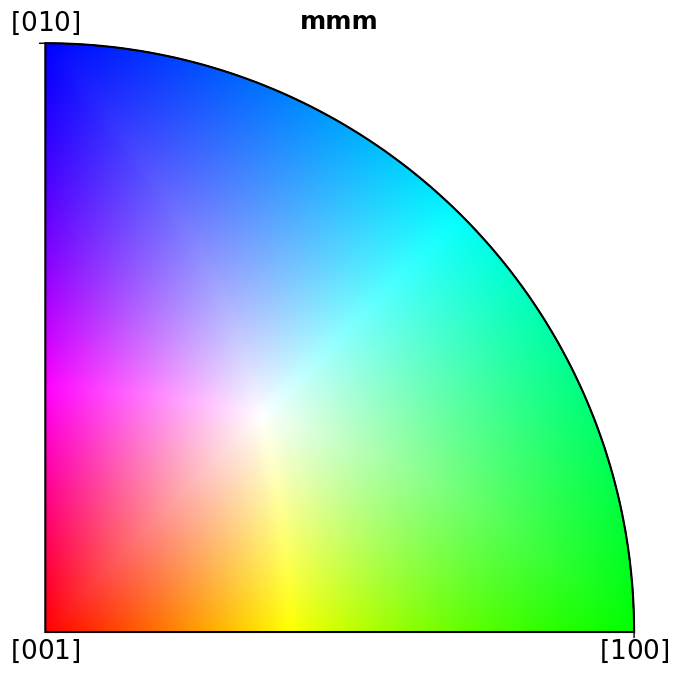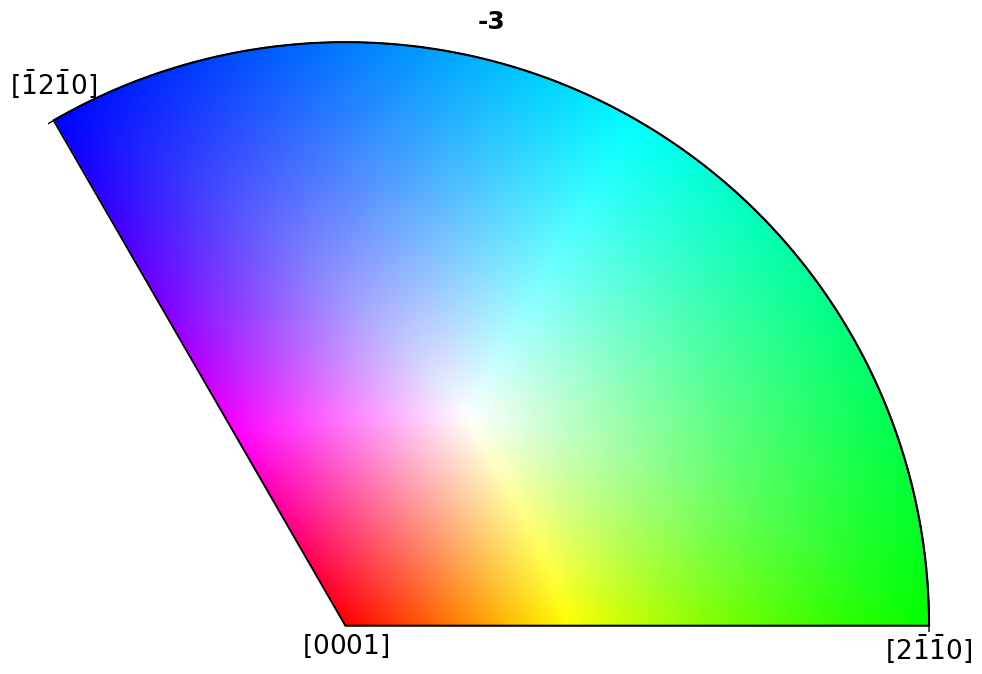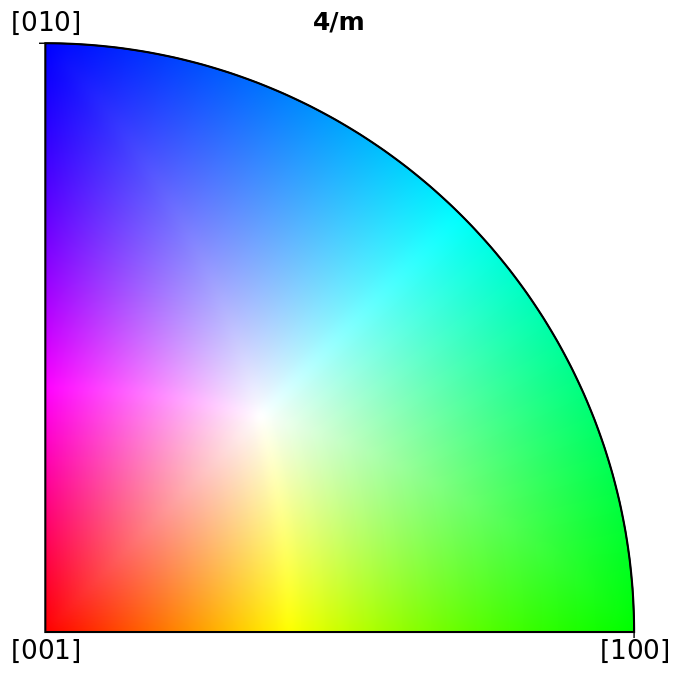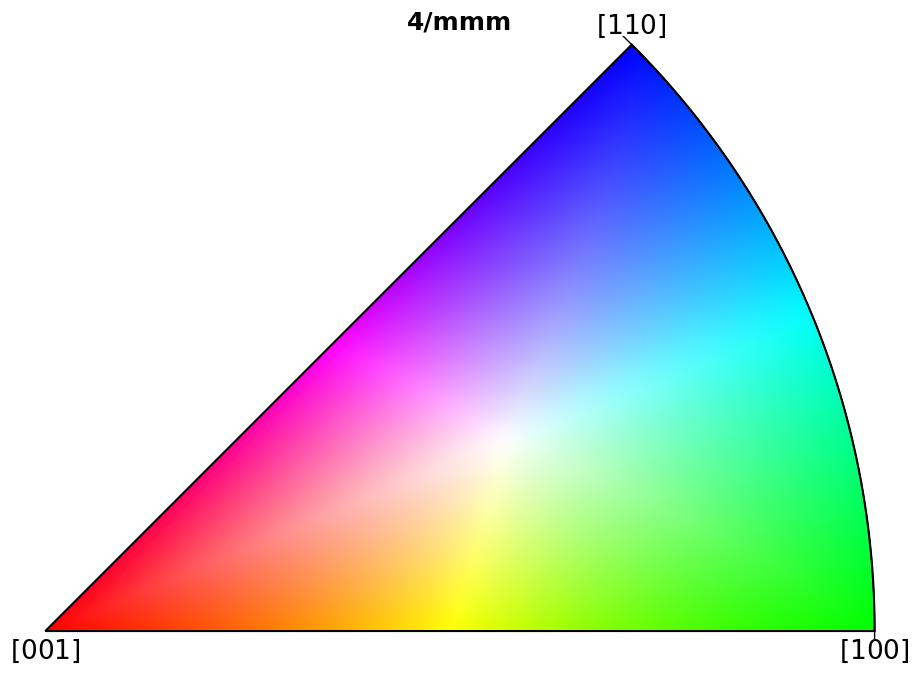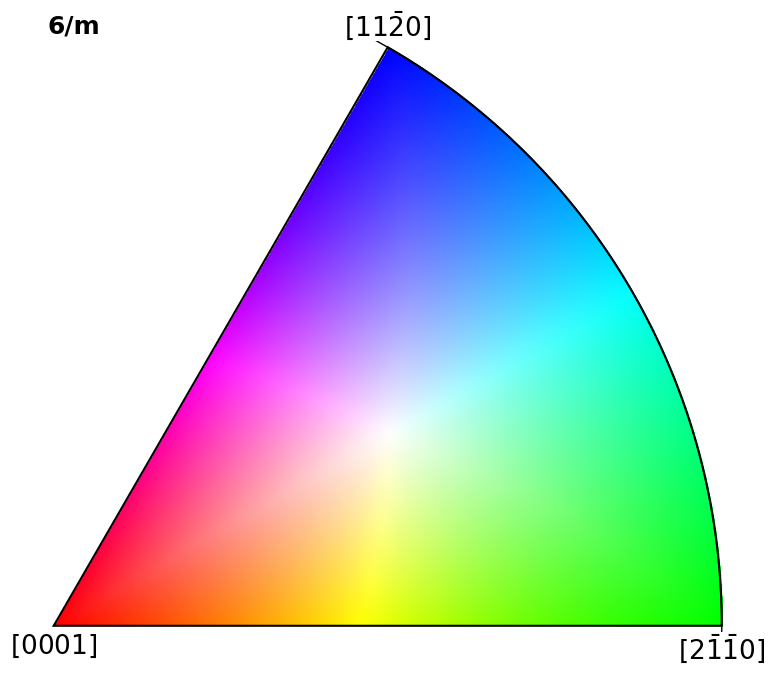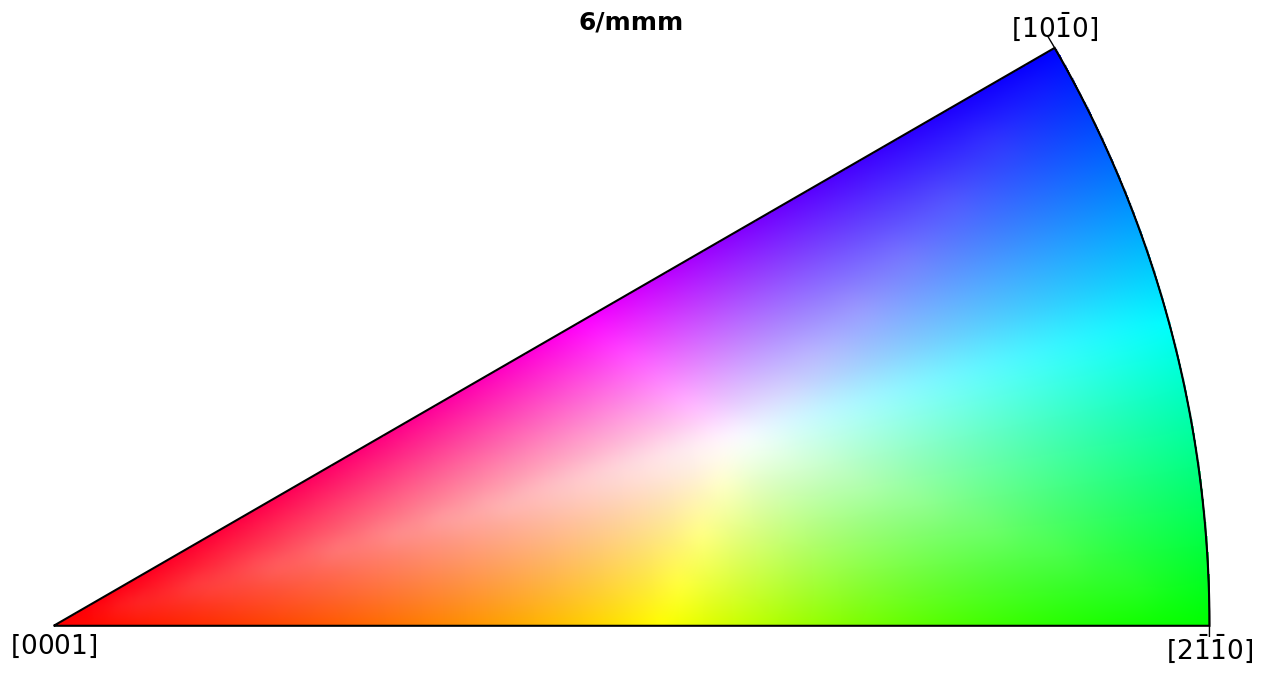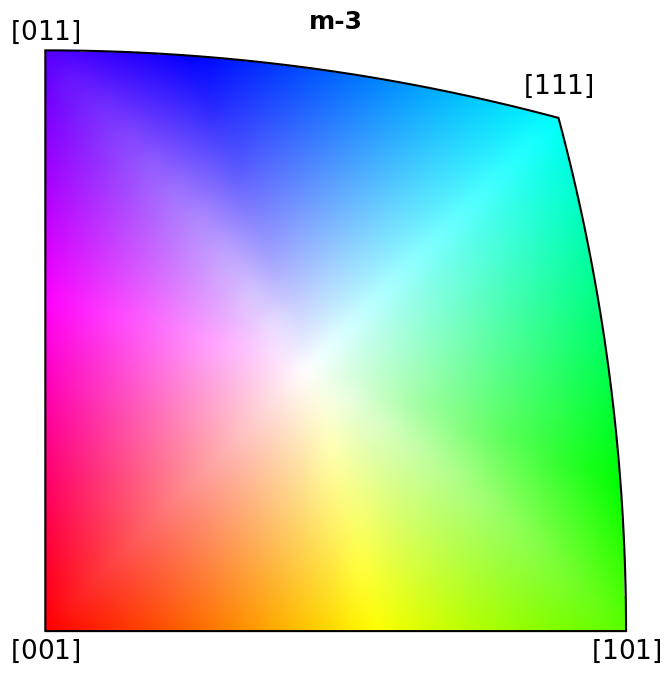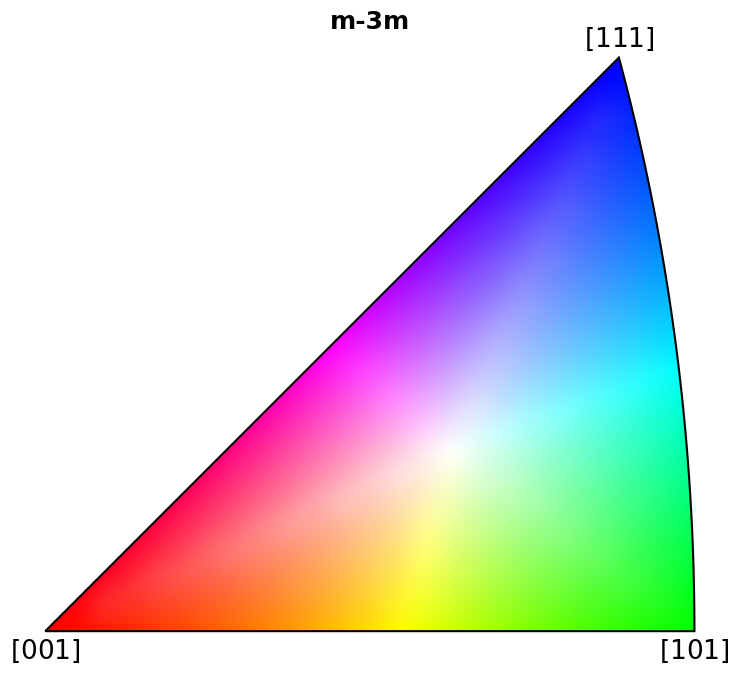## Scatter plot#

Here we show which crystal direction $$\left<uvw\right>$$ the sample reference frame directions X, Y, and Z point in. We can turn off the grid by updating the Matplotlib parameters with plt.rcParams["axes.grid"] = False, or passing grid=False to Orientation.scatter(), which we use to plot the IPFs.

:

direction = Vector3d(((1, 0, 0), (0, 1, 0), (0, 0, 1)))  # X, Y, Z
kwargs = dict(projection="ipf", direction=direction)

for pg in pg_laue:
ori = Orientation.from_euler([325, 48, 163], pg, degrees=True)
ori.scatter(**kwargs)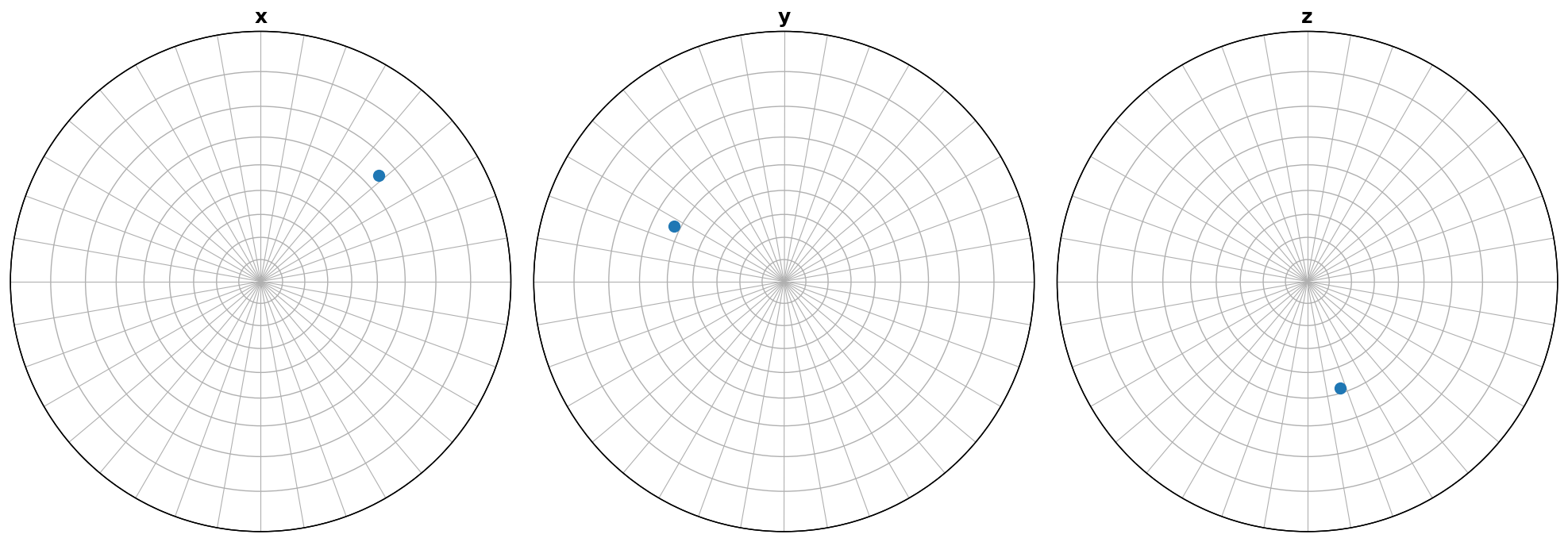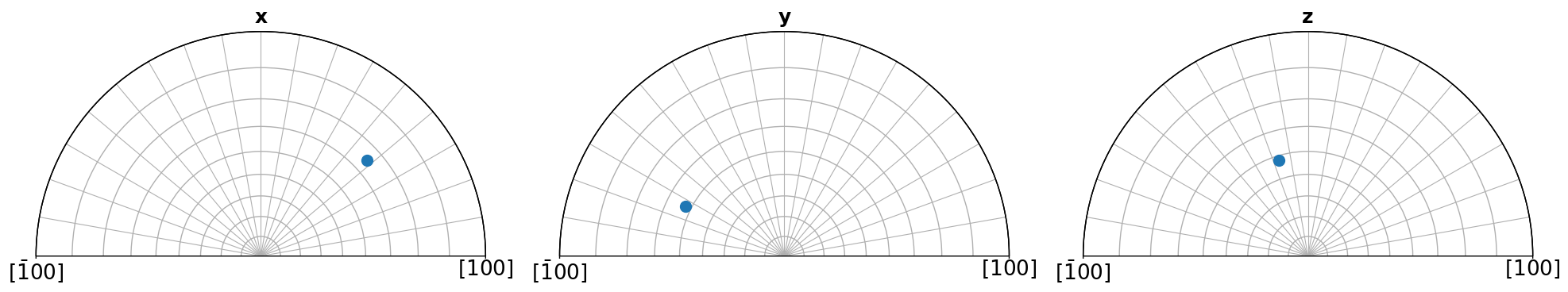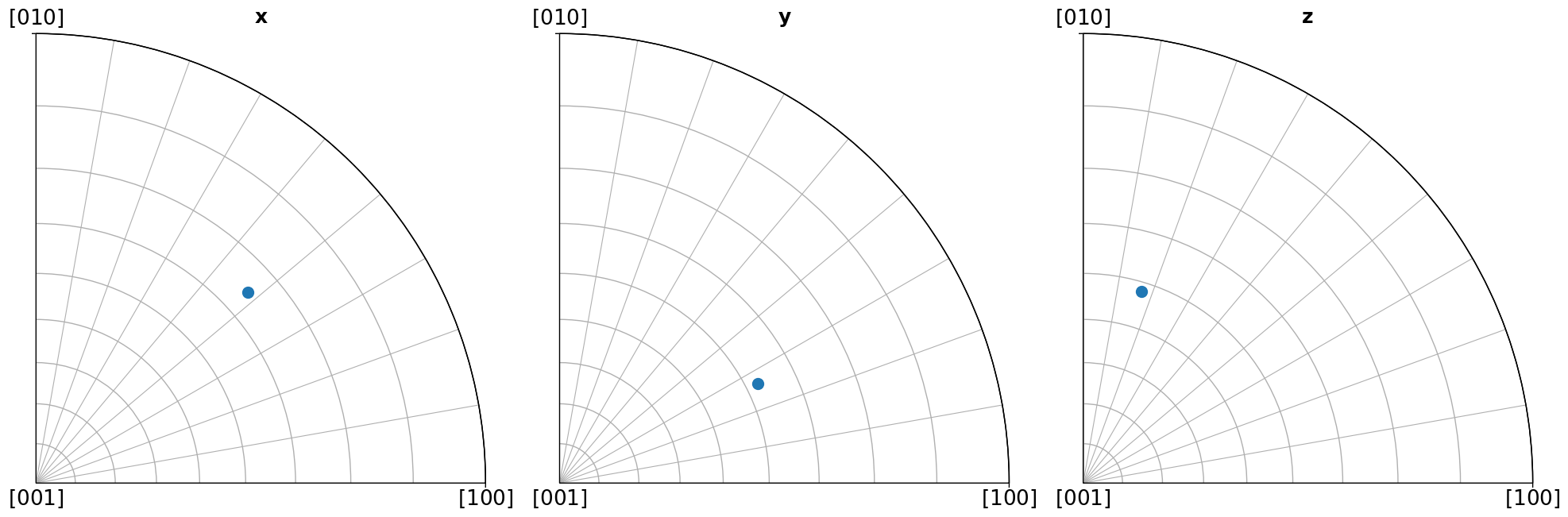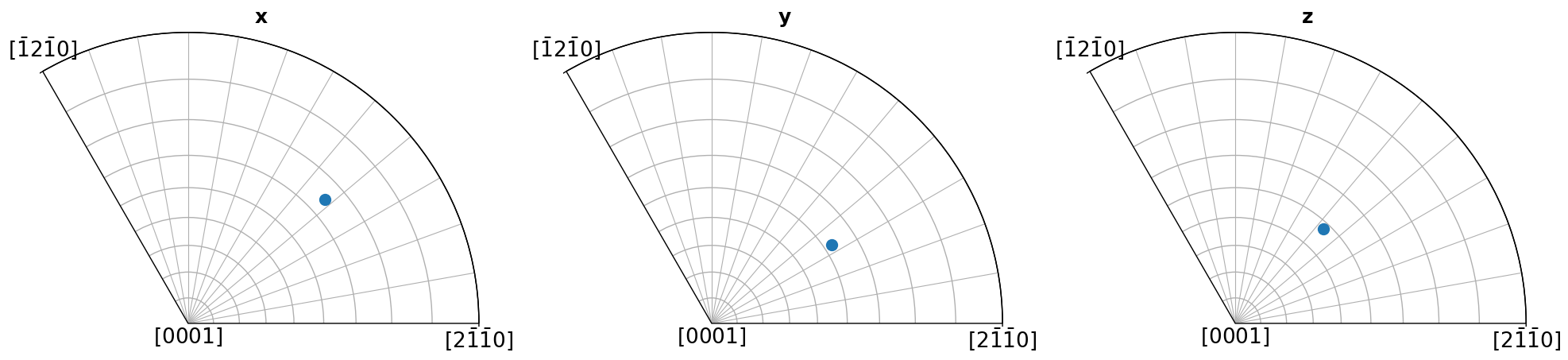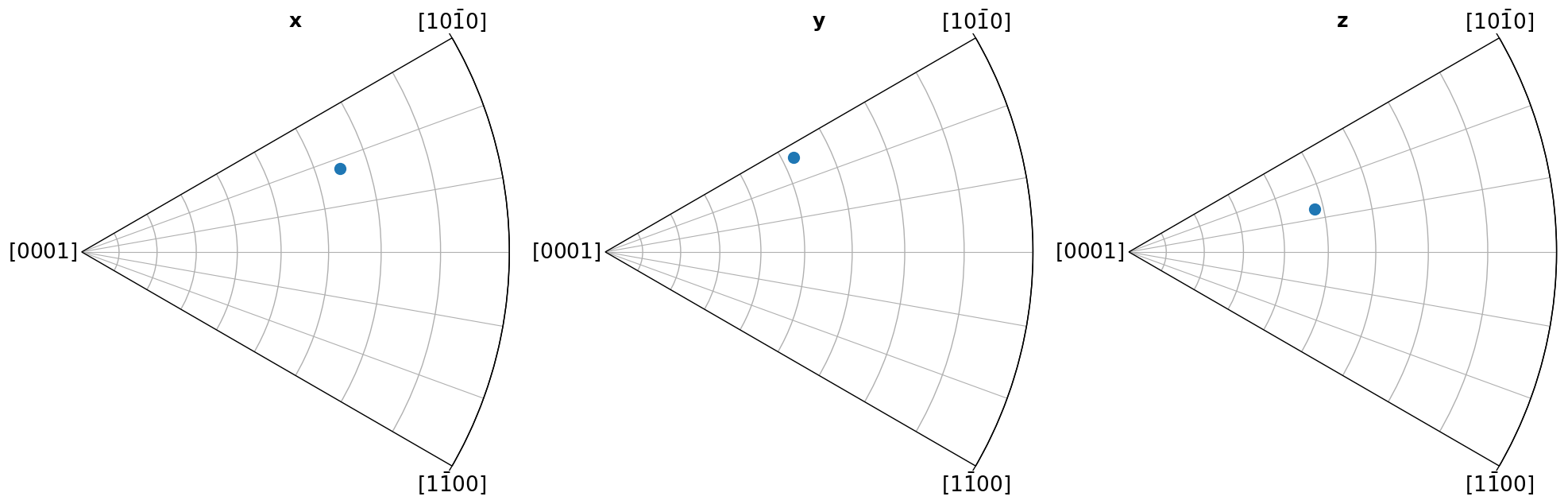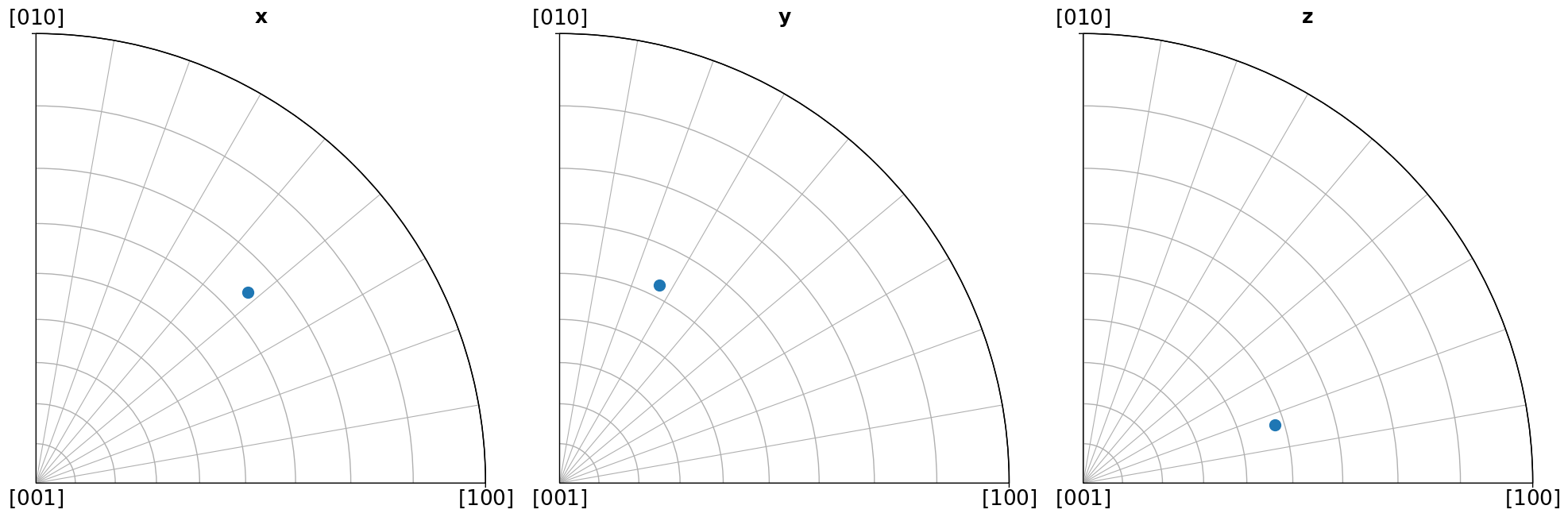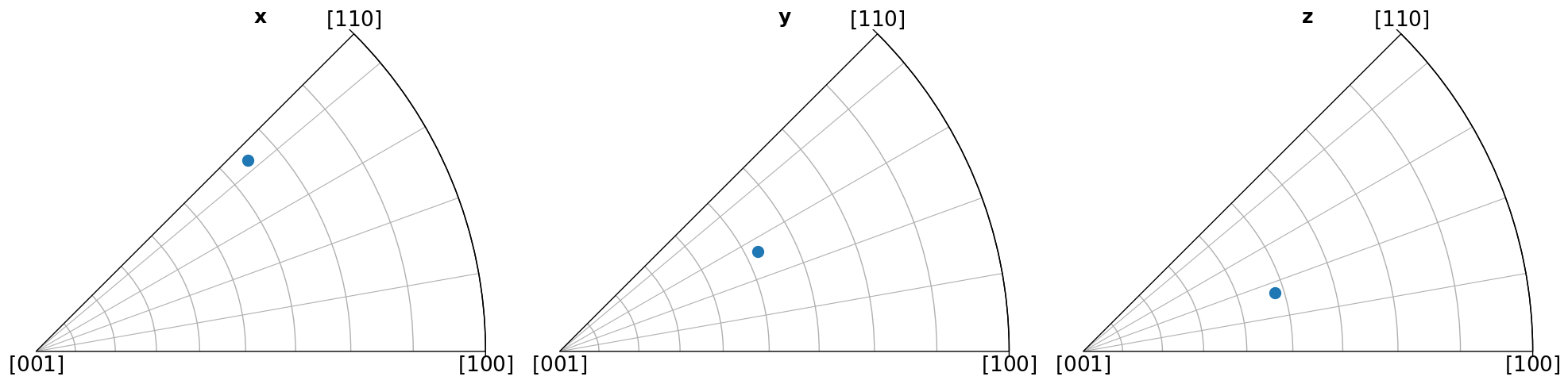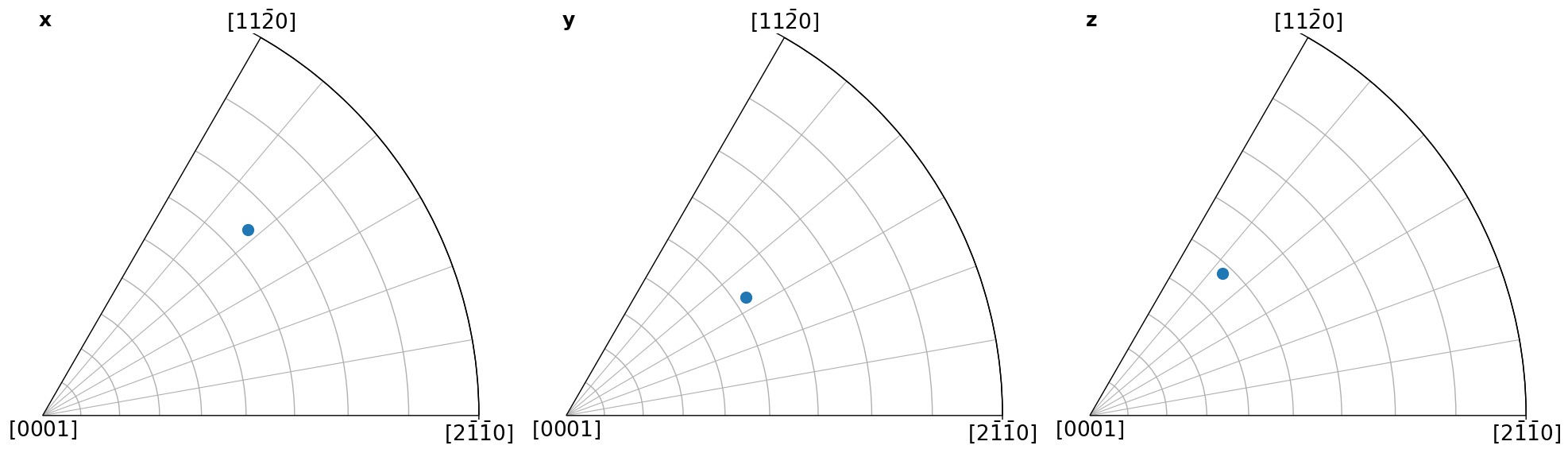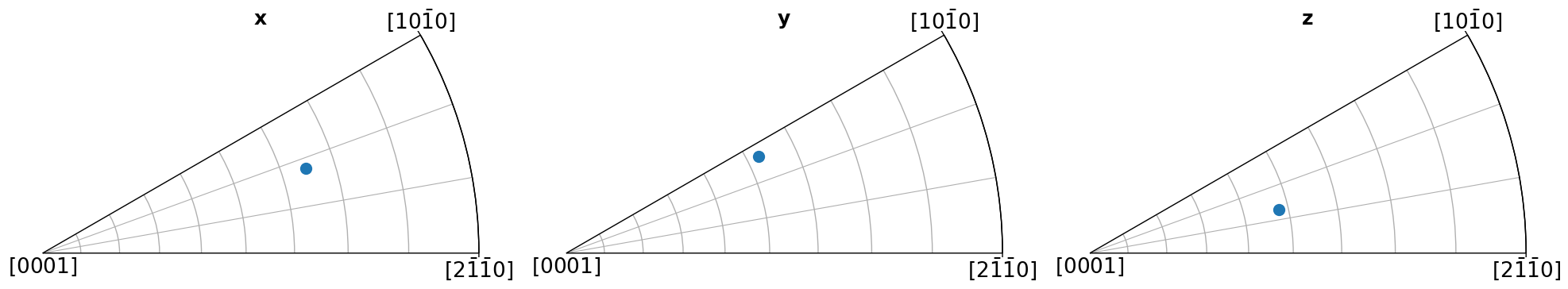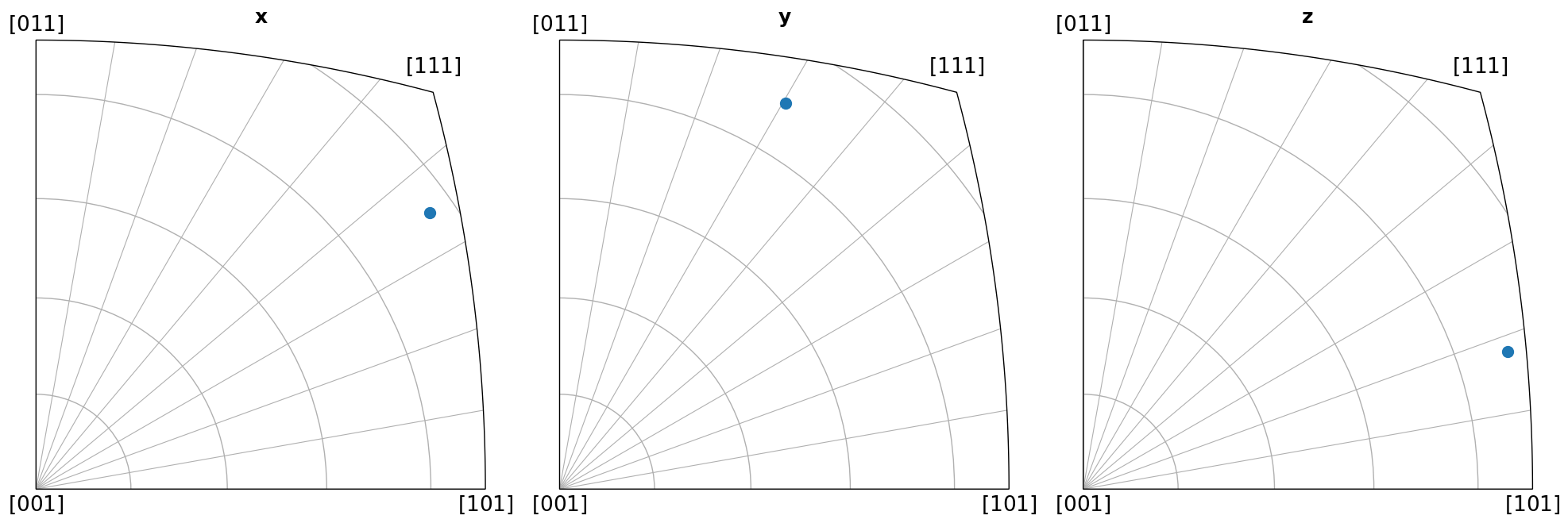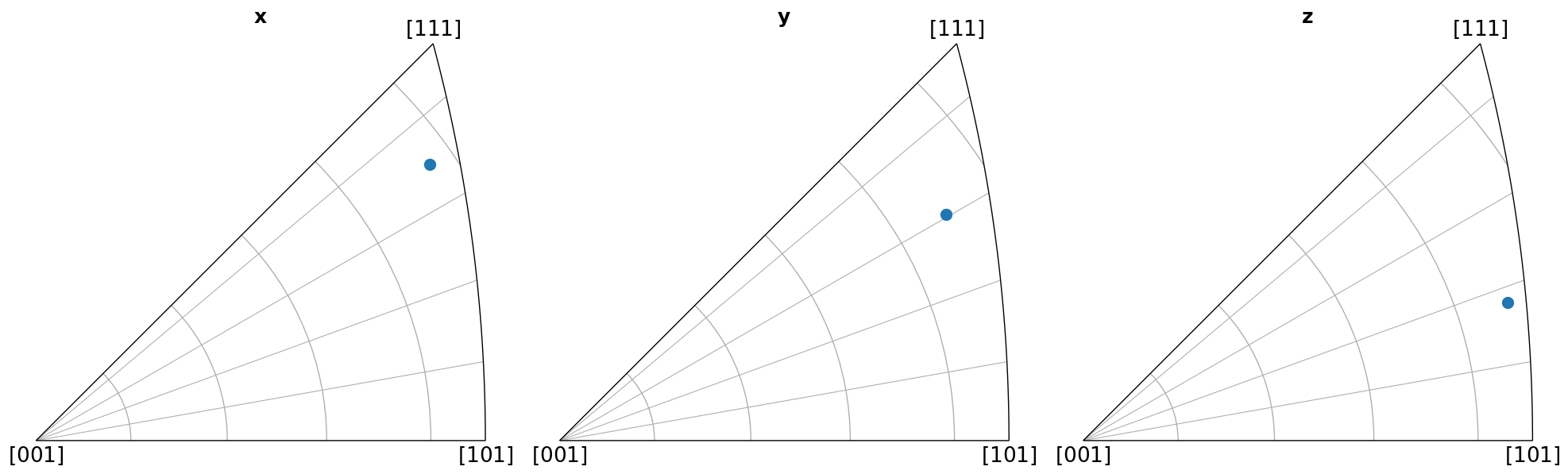## Colouring orientations#

Here we show how to obtain an RGB colour for each crystal orientation given a sample direction, using IPFColorKeyTSL.orientation2color. We then plot them in the IPF. Note that we could instead plot them in a map, say when the orientations come from a crystallographic mapping experiment. See the crystal map tutorial for an example.

:

plt.rcParams["axes.grid"] = False

ori2 = Orientation.random(1000)
for pg in pg_laue:
ipfkey = plot.IPFColorKeyTSL(pg)
ori2.symmetry = ipfkey.symmetry
rgb_z = ipfkey.orientation2color(ori2)
ori2.scatter("ipf", c=rgb_z, direction=ipfkey.direction)